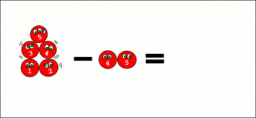# Calculate 64154

By rounding the number X = 47,546 to thousands, we get the number of hundreds, the number Y. Calculate X-Y.

r =  -454

### Step-by-step explanation:Did you find an error or inaccuracy? Feel free to write us. Thank you!

Tips for related online calculators
Do you want to round the number?